# User:IssaRice/Taking inf and sup separately

## Satement

Let$A$ and$B$ be bounded subsets of the real line. Suppose that for every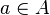$a\in A$ and$b\in B$ we have$a\geq b$. Then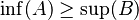$\inf(A)\geq \sup(B)$.

Actually, do$A$ and$B$ have to be bounded? I think they can even be empty!

## Proof

Let$a\in A$ and$b\in B$ be arbitrary. We have by hypothesis$a\geq b$. Since$b$ is arbitrary, we have that$a$ is an upper bound of the set$B$, so taking the superemum over$b$ we have$a \geq \sup(B)$ (remember,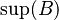$\sup(B)$ is the least upper bound, whereas$a$ is just another upper bound). Since$a$ was arbitrary, we see that$\sup(B)$ is a lower bound of the set$A$. Taking the infimum over$a$, we have$\inf(A) \geq \sup(B)$, as required.

## Applications

### liminf vs limsup

(Notation from Tao's Analysis I.)

Let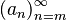$(a_n)_{n=m}^\infty$ be a sequence of real numbers. Let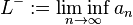$L^- := \liminf_{n\to\infty} a_n$ and let$L^+ := \limsup_{n\to\infty} a_n$. Then we have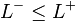$L^- \leq L^+$.

Consider the sequences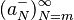$(a^-_N)_{N=m}^\infty$ and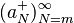$(a^+_N)_{N=m}^\infty$ defined by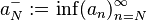$a^-_N := \inf(a_n)_{n=N}^\infty$ and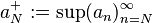$a^+_N := \sup(a_n)_{n=N}^\infty$.

Now consider the sets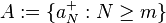$A := \{a^+_N : N \geq m\}$ and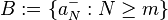$B := \{a^-_N : N \geq m\}$. If we can show that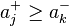$a^+_j \geq a^-_k$ for arbitrary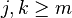$j,k\geq m$, then we can apply the trick to these sets to conclude that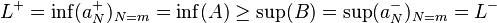$L^+ = \inf(a^+_N)_{N=m} = \inf(A) \geq \sup(B) = \sup(a^-_N)_{N=m} = L^-$.

### Lower and upper Riemann integral

(Notation from Tao's Analysis I.)

Let$I$ be a bounded interval on the real line, and let$f : I \to \mathbf R$.

We have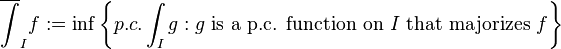$\overline{\int}_I f := \inf\left\{p.c.\int_I g : g\text{ is a p.c. function on }I\text{ that majorizes }f\right\}$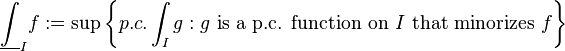$\underline{\int}_I f := \sup\left\{p.c.\int_I g : g\text{ is a p.c. function on }I\text{ that minorizes }f\right\}$

We want to show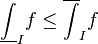$\underline{\int}_I f \leq \overline{\int}_I f$.

Define$A := \left\{p.c.\int_I g : g\text{ is a p.c. function on }I\text{ that majorizes }f\right\}$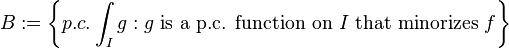$B := \left\{p.c.\int_I g : g\text{ is a p.c. function on }I\text{ that minorizes }f\right\}$

Then we have$\overline{\int}_I f = \inf(A)$ and$\underline{\int}_I f = \sup(B)$. To apply the trick all we need to do is to let$g$ be a p.c. function on$I$ that majorizes$f$, and let$h$ be a p.c. function on$I$ that minorizes$f$, and show that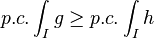$p.c.\int_I g\geq p.c.\int_I h$.

## alternating series test

(this one is more of a failed application)

each even partial sum is at least as large as each odd partial sum, so the inf over the even partial sums is at least as large as the sup over the odd partial sums. this actually isn't strong enough to prove what we want. we actually need the stronger condition that the even partial sums are a decreasing sequence, and that the odd partial sums are an increasing sequence, and that eventually their difference becomes arbitrarily small.